GeeksforGeeks App
Open AppBrowser
Continue

# Power BI – DAX Depreciation Functions

Formulas that do financial computations, including net present value and rate of return, use financial functions in DAX. These features are comparable to those found in Microsoft Excel’s financial features. Microsoft added about 50 additional DAX capabilities to Power BI in July 2020. Some users may already be familiar with these features since they are comparable to those in Excel. We intend to go over each of these features in a series of DAX Finance articles for people who are unfamiliar with them so that you can learn what they do and how to utilize them.

The depreciation functions will be covered in detail in the first installment of the DAX Finance series. Keep in mind that the return value for each depreciation function is only valid for the chosen period(s). It is simple to create accumulated depreciation and other derivative values, however, there are additional processes involved.

Dataset

Here, we are applying the functions on a sample superstore dataset. A screenshot of the same can be seen below: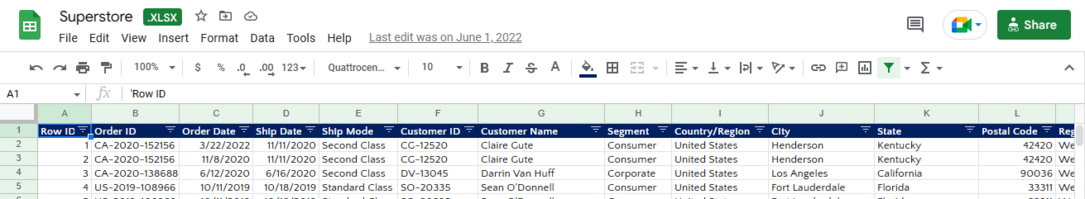### DAX Financial functions

The following DAX Financial functions are frequently used to return depreciation for a given period:

### DAX AMORDEGRC

The depreciation for each accounting period is returned. The French accounting system can use this feature. Prorated depreciation is considered when an asset is acquired in the midst of the accounting period. The function is comparable to AMORLINC with the exception that the calculation uses a depreciation coefficient based on the asset life.

Syntax: AMORDEGRC(<cost>, <date_purchased>, <first_period>, <salvage>, <period>, <rate>[, <basis>])

### Parameters

• Cost: The asset’s price.
• Date_purchased: The day the asset was purchased.
• First_period: The day that the first period ended.
• Salvage: The asset’s salvage value at the end of its useful life.
• Period: Time period.
• Rate: The depreciation rate.
• Basis: The kind of day count foundation to employ (optional). The basis is taken to be 0 if it is omitted. Below this table is a list of acceptable values.

### Output

Each accounting period’s depreciation.

Example: amordegrc = EVALUATEANDLOG(AMORDEGRC(2400, DATE(2008,8,19), DATE(2008,12,31), 300, 1, 0.15, 1))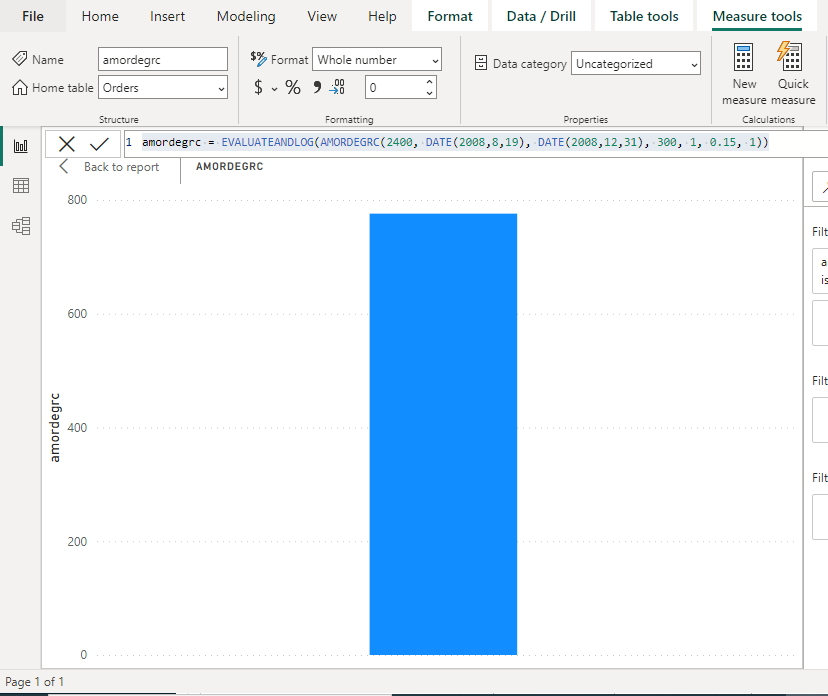Note:

In order to be used in calculations, dates are recorded as consecutive serial numbers. Since January 1, 2008, is 39,448 days after December 30, 1899, it is represented in the DAX as day 0, and December 30, 1899, as day 0. This function will return the depreciation up to the end of the asset’s life or until the total value of depreciation exceeds the asset’s purchase price less salvage value. The coefficients of depreciation are:

The depreciation rate will increase to 50% for the period immediately before the last period and to 100% for the final period. The nearest integer is used to round the period and basis. A failure is indicated if

• Cost < 0.
• Neither the first period nor the date purchased is a valid date.
• date purchased > first-period
• salvage 0 or salvage > for the first period Cost.
• Duration < 0.
• Rate<= 0.

Assets have a life between zero (zero) and one, one and two, two and three, or four and five. The basis is any number other than zero, one, three, or four. In calculated columns or with RLS rules, this function is not supported for use in Direct Query mode.

### DAX AMORLINC

The depreciation for each accounting period is returned. The French accounting system can use this feature. Prorated depreciation is considered when an asset is acquired in the midst of the accounting period.

Syntax: AMORLINC(<cost>, <date_purchased>, <first_period>, <salvage>, <period>, <rate>[, <basis>])

### Parameters

• Cost: The asset’s price.
• Date_purchased: The day the asset was purchased.
• First_period: The day that the first period ended.
• salvage: The asset’s salvage value at the end of its useful life.
• Period: Time period.
• Rate: The rate depreciation rate.
• Basis: The kind of day count foundation to employ (optional). The basis is taken to be 0 if it is omitted. Below this table is a list of acceptable values.

### Output

Each accounting period’s depreciation.

Example: amorlinc = EVALUATEANDLOG(AMORLINC(2400, DATE(2008,8,19), DATE(2008,12,31), 300, 1, 0.15, 1))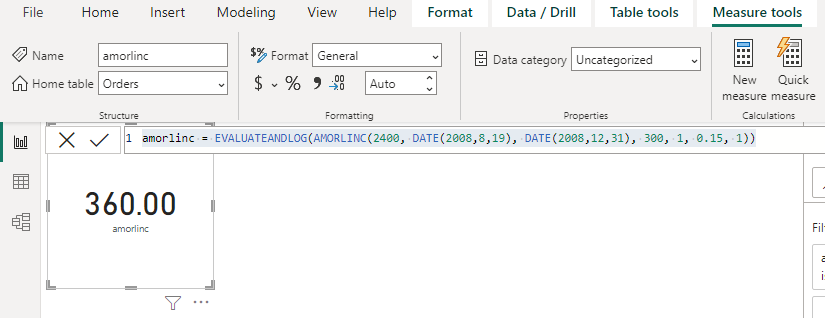Note:

In order to be used in calculations, dates are recorded as consecutive serial numbers. Since January 1, 2008, is 39,448 days after December 30, 1899, it is represented in the DAX as day 0, and December 30, 1899, as day 0. The nearest integer is used to round the period and basis. A failure is indicated if,

• Cost < 0.
• Neither the first period nor the date purchased is a valid date.
• date purchased > first-period
• salvage 0 or salvage > for the first period Cost.
• Duration < 0.
• Rate<= 0.

Assets have a life between zero (zero) and one, one and two, two and three, or four and five. The basis is any number other than zero, one, three, or four. In calculated columns or with RLS rules, this function is not supported for use in Direct Query mode.

### DAX DB

DAX DB gives back an asset’s depreciation over a given period using the fixed-declining balanced approach.

Syntax: DB(<cost>, <salvage>, <life>, <period>[, <month>])

### Parameters

• Cost: The asset’s initial price.
• Salvage: The final value after depreciation (sometimes called the salvage value of the asset). This number may be zero.
• Life: The length of time that an asset is depreciated (sometimes called the useful life of the asset).
• Period: The time frame that you want to use to determine depreciation. The same units must be used for a period and life. between 1 and life, please (inclusive).
• Month: The total number of months in the first year, if applicable. A month is taken to be 12 if it is absent.

### Output

The depreciation over the predetermined time.

Example: db = EVALUATEANDLOG(DB(1000000, 0, 6, 1, 2))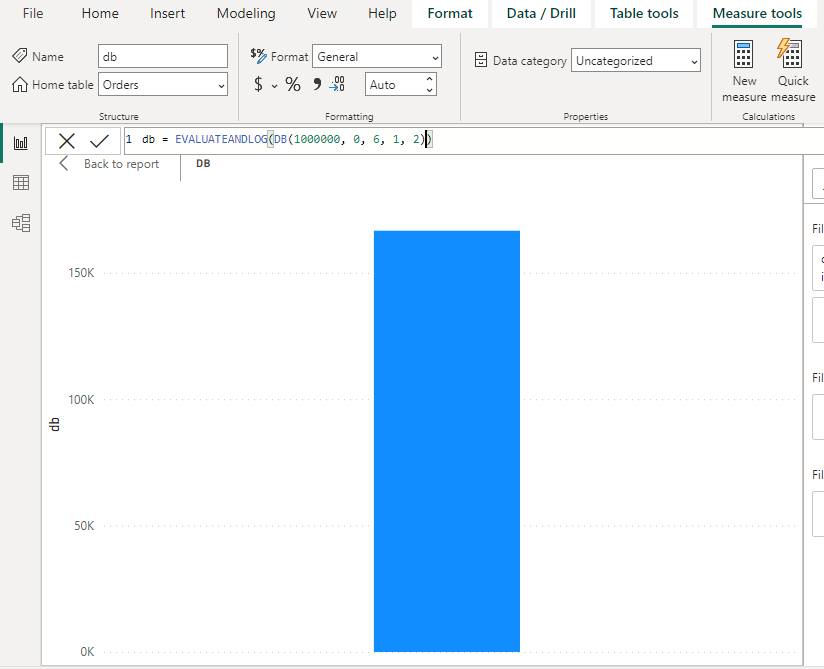Note:

Depreciation is calculated using the fixed-declining balance approach at a fixed rate. DB computes depreciation for a period using the following formulas:

(Cost – Total depreciation from prior periods) × rate

Where,Rounded to three decimal places. Depreciation for the initial and final periods is a unique situation.

• DB applies the following formula to the first period ⇢ [cost × rate × month]/12
• DB applies the following formula to the previous period: [(cost – total depreciation from prior periods) × rate × (12 – month)]/12

Month and period are rounded to the nearest whole number. A failure is indicated if,

• Cost < 0.
• Salvage < 0.
• Life < 1.
• Period < 1 or period > life.
• Month < 1 or month > 12.

In calculated columns or with RLS rules, this function is not supported for use in Direct Query mode.

### DAX DDB

This function returns the depreciation of an asset for a specified period using the double-declining balance method or some other method you specify.

Syntax: DDB(<cost>, <salvage>, <life>, <period>[, <factor>])

### Parameters

• Cost: The asset’s initial price.
• Salvage: The final value after depreciation (sometimes called the salvage value of the asset). This number may be zero.
• Life: The length of time that an asset is depreciated (sometimes called the useful life of the asset).
• Period: The time frame that you want to use to determine depreciation. The same units must be used for a period and life. Between 1 and life (inclusive).
• Factor: The rate at which the balance is losing value (optional). The factor is taken to be 2 if it is missing (the double-declining balance method).

### Output

The depreciation over the predetermined time.

Example: ddb = EVALUATEANDLOG(DDB(1000000, 0, 6, 1, 2))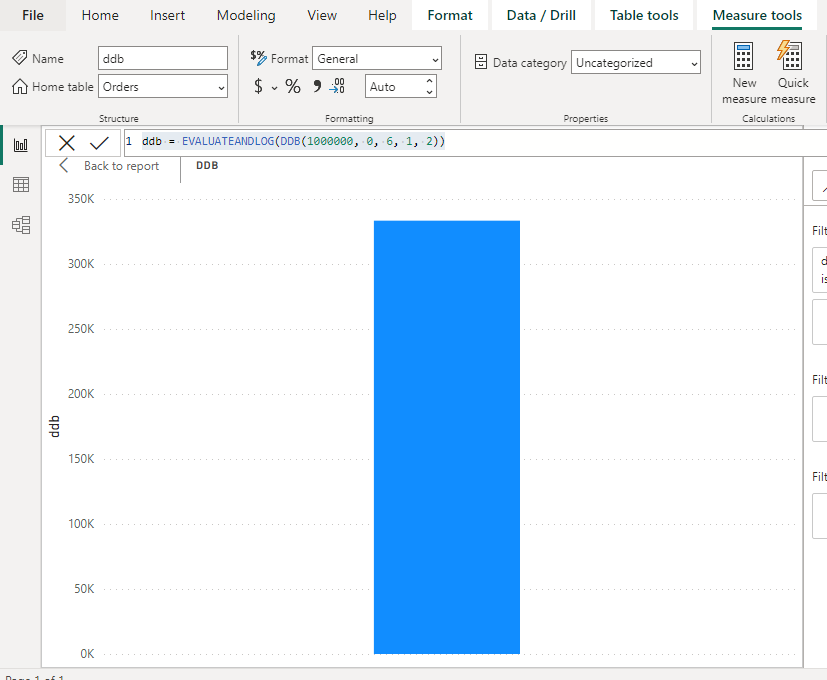Note:

The double-declining balance method accelerates the calculation of depreciation. The rate of depreciation is largest during the initial period and gets lower as time goes on. DDB calculates depreciation for a period using the following formula:

Min[(cost – total depreciation from prior periods) × (factorlife), (cost – salvage – total depreciation from prior periods)]

If you don’t want to utilize the double-declining balance method, change the factor. When depreciation exceeds the decreasing balance computation and you want to switch to the straight-line depreciation technique, use the VDB function. The period is rounded to the closest whole number. A failure is indicated if,

• Cost < 0.
• Salvage < 0.
• Life < 1.
• Period < 1 or period > life.
• Factor ≤ 0.

In calculated columns or with RLS rules, this function is not supported for use in Direct Query mode.

### DAX SLN

DAX SLN returns the straight-line depreciation of an asset for one period.

Syntax: SLN(<cost>, <salvage>, <life>)

### Parameters

• Cost- The asset’s initial price.
• Salvage- The final value after depreciation (sometimes called the salvage value of the asset). This number may be zero.
• Life- The length of time that an asset is depreciated (sometimes called the useful life of the asset).

### Output

The one-period straight-line depreciation.

Example: sln = EVALUATEANDLOG(SLN(30000, 7500, 10))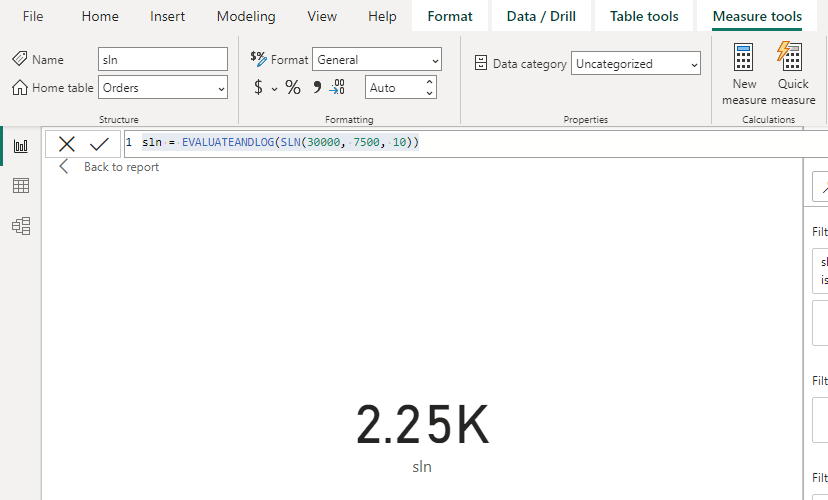Note:

A failure is indicated if, life = 0. This function is not supported for use in Direct Query mode when used in calculated columns or row-level security (RLS) rules.

### DAX SYD

It returns the sum-of-years digits depreciation of an asset for a specified period.

Syntax: SYD(<cost>, <salvage>, <life>, <per>)

### Parameters

• Cost: The asset’s initial price.
• Salvage: The final value after depreciation (sometimes called the salvage value of the asset). This number may be zero.
• Life: The length of time that an asset is depreciated (sometimes called the useful life of the asset).
• Per: A time frame. must utilize life-like units. between 1 and life, please (inclusive).

### Output

Depreciation during the given time period is expressed as the sum of years’ digits.

Example: syd = EVALUATEANDLOG( SYD(30000.00, 7500.00, 10, 1))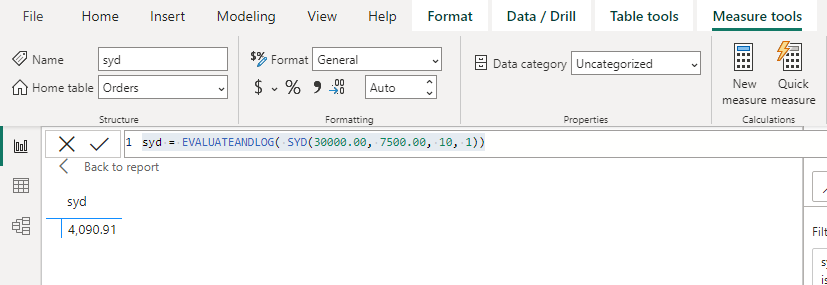Note:

The formula for SYD is as follows:A failure is indicated if,

life < 1.
per < 1 or per > life.

In calculated columns or with RLS rules, this function is not supported for use in Direct Query mode.

My Personal Notes arrow_drop_up